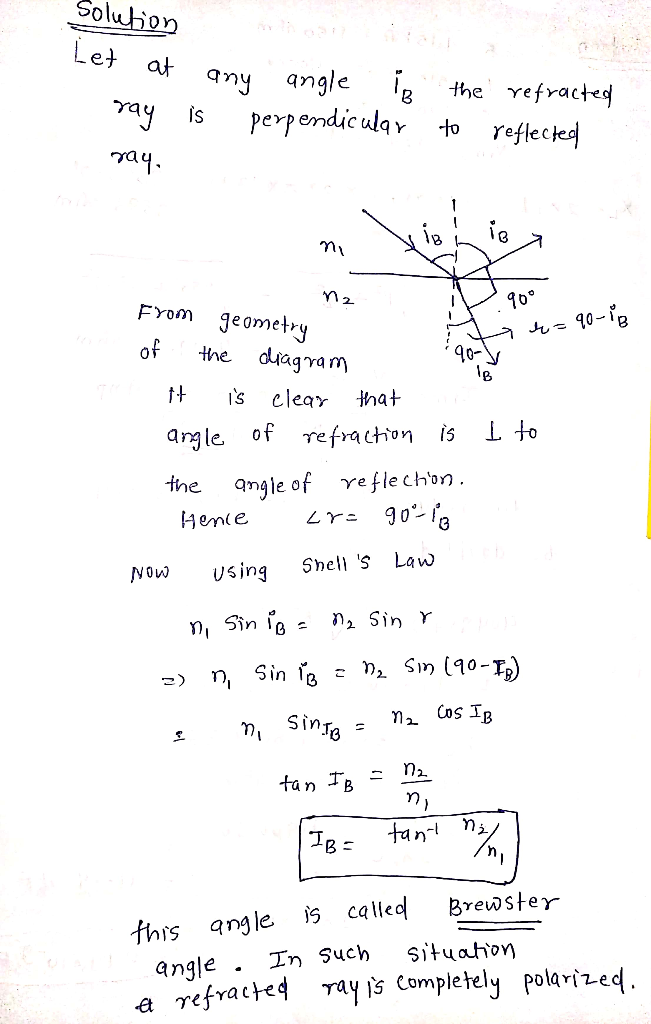In: Physics

# When reaching a boundary between two media, an incident ray is partially reflected and partially refracted....

When reaching a boundary between two media, an incident ray is partially reflected and partially refracted. The ray is travelling from media 1 to media 2. The indexes of refraction for the two media are n1 and n2, respectively. At what angle of incidence is the reflected ray perpendicular to the refracted ray? Express your answer in terms of the variables n1 and n2.

## Solutions

##### Expert Solution## Related Solutions

##### A light ray strikes the perfectly smooth boundary between two different materials. The index of refraction...
A light ray strikes the perfectly smooth boundary between two different materials. The index of refraction of the material before the boundary is lower than that of the material after the boundary. On the basis of this information, the light ray will be a. partially transmitted in a direction deviating more from the normal and partially reflected. b. partially transmitted in a direction deviating less from the normal and partially reflected. c. partially transmitted exactly in the same direction and...
##### ​A ray of light is incident onto the interface between material 1 and material 2.
A ray of light is incident onto the interface between material 1 and material 2.Given the indices of refraction n_1 and n_2 of material 1 and material 2, respectively, rank these scenarios on the basis of the phase shift in the refracted ray.1) Rank from largest to smallest. To rank items as equivalent, overlap them.a) n1,water=1.33n2,air=1.00b) n1,water=1.33n2,quartz=1.46c) n1,water=1.33n2,diamond=2.42d) n1,air=1.00n2,quartz=1.46e) n1,benzene=1.50n2,water=1.33f) n1,air=1.00n2,water=1.332) Rank these scenarios on the basis of the phase shift in the reflected ray.a) n1,benzene=1.50n2,water=1.33b) n1,air=1.00n2,quartz=1.46c) n1,water=1.33n2,quartz=1.46d) n1,water=1.33n2,air=1.00e) n1,water=1.33n2,diamond=2.42f)...
##### Name two differences between an XPS X-Ray source and an XRD X-Ray source. Explain why they...
Name two differences between an XPS X-Ray source and an XRD X-Ray source. Explain why they are different.
##### An earthquake P wave traveling at 7.7km/s strikes a boundary within the Earth between two kinds...
An earthquake P wave traveling at 7.7km/s strikes a boundary within the Earth between two kinds of material. If it approaches the boundary at an incident angle of 44? and the angle of refraction is 33?, what is the speed in the second medium? Express your answer to two significant figures and include the appropriate units.
##### Which of the following is least likely to decrease the thermal contact resistance between two media...
Which of the following is least likely to decrease the thermal contact resistance between two media in contact? Make a vacuum gap at the interface Apply thermal grease onto the interface Sand the contact surface smooth Apply pressure one against another